Help protect the Great Barrier Reef with TensorFlow on Kaggle

Ragged tensors

API Documentation: tf.RaggedTensor tf.ragged

Setup

import math
import tensorflow as tf

Overview

Your data comes in many shapes; your tensors should too. Ragged tensors are the TensorFlow equivalent of nested variable-length lists. They make it easy to store and process data with non-uniform shapes, including:

• Variable-length features, such as the set of actors in a movie.
• Batches of variable-length sequential inputs, such as sentences or video clips.
• Hierarchical inputs, such as text documents that are subdivided into sections, paragraphs, sentences, and words.
• Individual fields in structured inputs, such as protocol buffers.

What you can do with a ragged tensor

Ragged tensors are supported by more than a hundred TensorFlow operations, including math operations (such as tf.add and tf.reduce_mean), array operations (such as tf.concat and tf.tile), string manipulation ops (such as tf.substr), control flow operations (such as tf.while_loop and tf.map_fn), and many others:

digits = tf.ragged.constant([[3, 1, 4, 1], [], [5, 9, 2], , []])
words = tf.ragged.constant([["So", "long"], ["thanks", "for", "all", "the", "fish"]])
print(tf.reduce_mean(digits, axis=1))
print(tf.concat([digits, [[5, 3]]], axis=0))
print(tf.tile(digits, [1, 2]))
print(tf.strings.substr(words, 0, 2))
print(tf.map_fn(tf.math.square, digits))
<tf.RaggedTensor [[6, 4, 7, 4], [], [8, 12, 5], , []]>
tf.Tensor([2.25              nan 5.33333333 6.                nan], shape=(5,), dtype=float64)
<tf.RaggedTensor [[3, 1, 4, 1], [], [5, 9, 2], , [], [5, 3]]>
<tf.RaggedTensor [[3, 1, 4, 1, 3, 1, 4, 1], [], [5, 9, 2, 5, 9, 2], [6, 6], []]>
<tf.RaggedTensor [[b'So', b'lo'], [b'th', b'fo', b'al', b'th', b'fi']]>
<tf.RaggedTensor [[9, 1, 16, 1], [], [25, 81, 4], , []]>

There are also a number of methods and operations that are specific to ragged tensors, including factory methods, conversion methods, and value-mapping operations. For a list of supported ops, see the tf.ragged package documentation.

Ragged tensors are supported by many TensorFlow APIs, including Keras, Datasets, tf.function, SavedModels, and tf.Example. For more information, check the section on TensorFlow APIs below.

As with normal tensors, you can use Python-style indexing to access specific slices of a ragged tensor. For more information, refer to the section on Indexing below.

print(digits)       # First row
tf.Tensor([3 1 4 1], shape=(4,), dtype=int32)
print(digits[:, :2])   # First two values in each row.
<tf.RaggedTensor [[3, 1], [], [5, 9], , []]>
print(digits[:, -2:])  # Last two values in each row.
<tf.RaggedTensor [[4, 1], [], [9, 2], , []]>

And just like normal tensors, you can use Python arithmetic and comparison operators to perform elementwise operations. For more information, check the section on Overloaded operators below.

print(digits + 3)
<tf.RaggedTensor [[6, 4, 7, 4], [], [8, 12, 5], , []]>
print(digits + tf.ragged.constant([[1, 2, 3, 4], [], [5, 6, 7], , []]))
<tf.RaggedTensor [[4, 3, 7, 5], [], [10, 15, 9], , []]>

If you need to perform an elementwise transformation to the values of a RaggedTensor, you can use tf.ragged.map_flat_values, which takes a function plus one or more arguments, and applies the function to transform the RaggedTensor's values.

times_two_plus_one = lambda x: x * 2 + 1
print(tf.ragged.map_flat_values(times_two_plus_one, digits))
<tf.RaggedTensor [[7, 3, 9, 3], [], [11, 19, 5], , []]>

Ragged tensors can be converted to nested Python lists and NumPy arrays:

digits.to_list()
[[3, 1, 4, 1], [], [5, 9, 2], , []]
digits.numpy()
/tmpfs/src/tf_docs_env/lib/python3.7/site-packages/tensorflow/python/ops/ragged/ragged_tensor.py:2063: VisibleDeprecationWarning: Creating an ndarray from ragged nested sequences (which is a list-or-tuple of lists-or-tuples-or ndarrays with different lengths or shapes) is deprecated. If you meant to do this, you must specify 'dtype=object' when creating the ndarray
return np.array(rows)
array([array([3, 1, 4, 1], dtype=int32), array([], dtype=int32),
array([5, 9, 2], dtype=int32), array(, dtype=int32),
array([], dtype=int32)], dtype=object)

Constructing a ragged tensor

The simplest way to construct a ragged tensor is using tf.ragged.constant, which builds the RaggedTensor corresponding to a given nested Python list or NumPy array:

sentences = tf.ragged.constant([
["Let's", "build", "some", "ragged", "tensors", "!"],
["We", "can", "use", "tf.ragged.constant", "."]])
print(sentences)
<tf.RaggedTensor [[b"Let's", b'build', b'some', b'ragged', b'tensors', b'!'], [b'We', b'can', b'use', b'tf.ragged.constant', b'.']]>
paragraphs = tf.ragged.constant([
[['I', 'have', 'a', 'cat'], ['His', 'name', 'is', 'Mat']],
[['Do', 'you', 'want', 'to', 'come', 'visit'], ["I'm", 'free', 'tomorrow']],
])
print(paragraphs)
<tf.RaggedTensor [[[b'I', b'have', b'a', b'cat'], [b'His', b'name', b'is', b'Mat']], [[b'Do', b'you', b'want', b'to', b'come', b'visit'], [b"I'm", b'free', b'tomorrow']]]>

Ragged tensors can also be constructed by pairing flat values tensors with row-partitioning tensors indicating how those values should be divided into rows, using factory classmethods such as tf.RaggedTensor.from_value_rowids, tf.RaggedTensor.from_row_lengths, and tf.RaggedTensor.from_row_splits.

tf.RaggedTensor.from_value_rowids

If you know which row each value belongs to, then you can build a RaggedTensor using a value_rowids row-partitioning tensor: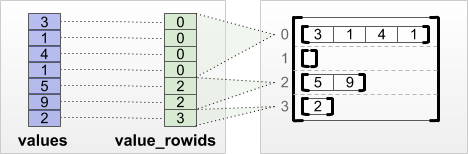print(tf.RaggedTensor.from_value_rowids(
values=[3, 1, 4, 1, 5, 9, 2],
value_rowids=[0, 0, 0, 0, 2, 2, 3]))
<tf.RaggedTensor [[3, 1, 4, 1], [], [5, 9], ]>

tf.RaggedTensor.from_row_lengths

If you know how long each row is, then you can use a row_lengths row-partitioning tensor: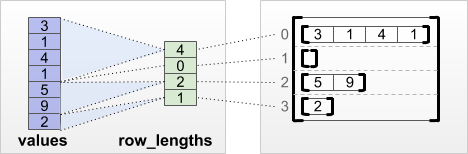print(tf.RaggedTensor.from_row_lengths(
values=[3, 1, 4, 1, 5, 9, 2],
row_lengths=[4, 0, 2, 1]))
<tf.RaggedTensor [[3, 1, 4, 1], [], [5, 9], ]>

tf.RaggedTensor.from_row_splits

If you know the index where each row starts and ends, then you can use a row_splits row-partitioning tensor: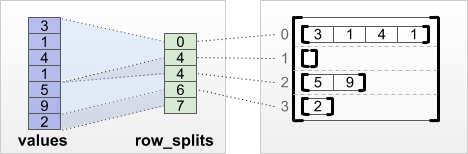print(tf.RaggedTensor.from_row_splits(
values=[3, 1, 4, 1, 5, 9, 2],
row_splits=[0, 4, 4, 6, 7]))
<tf.RaggedTensor [[3, 1, 4, 1], [], [5, 9], ]>

See the tf.RaggedTensor class documentation for a full list of factory methods.

What you can store in a ragged tensor

As with normal Tensors, the values in a RaggedTensor must all have the same type; and the values must all be at the same nesting depth (the rank of the tensor):

print(tf.ragged.constant([["Hi"], ["How", "are", "you"]]))  # ok: type=string, rank=2
<tf.RaggedTensor [[b'Hi'], [b'How', b'are', b'you']]>
print(tf.ragged.constant([[[1, 2], ], [[4, 5]]]))        # ok: type=int32, rank=3
<tf.RaggedTensor [[[1, 2], ], [[4, 5]]]>
try:
tf.ragged.constant([["one", "two"], [3, 4]])              # bad: multiple types
except ValueError as exception:
print(exception)
Can't convert Python sequence with mixed types to Tensor.
try:
tf.ragged.constant(["A", ["B", "C"]])                     # bad: multiple nesting depths
except ValueError as exception:
print(exception)
all scalar values must have the same nesting depth

Example use case

The following example demonstrates how RaggedTensors can be used to construct and combine unigram and bigram embeddings for a batch of variable-length queries, using special markers for the beginning and end of each sentence. For more details on the ops used in this example, check the tf.ragged package documentation.

queries = tf.ragged.constant([['Who', 'is', 'Dan', 'Smith'],
['Pause'],
['Will', 'it', 'rain', 'later', 'today']])

# Create an embedding table.
num_buckets = 1024
embedding_size = 4
embedding_table = tf.Variable(
tf.random.truncated_normal([num_buckets, embedding_size],
stddev=1.0 / math.sqrt(embedding_size)))

# Look up the embedding for each word.
word_buckets = tf.strings.to_hash_bucket_fast(queries, num_buckets)
word_embeddings = tf.nn.embedding_lookup(embedding_table, word_buckets)     # ①

# Add markers to the beginning and end of each sentence.
marker = tf.fill([queries.nrows(), 1], '#')
padded = tf.concat([marker, queries, marker], axis=1)                       # ②

# Build word bigrams and look up embeddings.

bigram_buckets = tf.strings.to_hash_bucket_fast(bigrams, num_buckets)
bigram_embeddings = tf.nn.embedding_lookup(embedding_table, bigram_buckets) # ④

# Find the average embedding for each sentence
all_embeddings = tf.concat([word_embeddings, bigram_embeddings], axis=1)    # ⑤
avg_embedding = tf.reduce_mean(all_embeddings, axis=1)                      # ⑥
print(avg_embedding)
tf.Tensor(
[[-0.14285272  0.02908629 -0.16327512 -0.14529026]
[-0.4479212  -0.35615516  0.17110227  0.2522229 ]
[-0.1987868  -0.13152348 -0.0325102   0.02125177]], shape=(3, 4), dtype=float32)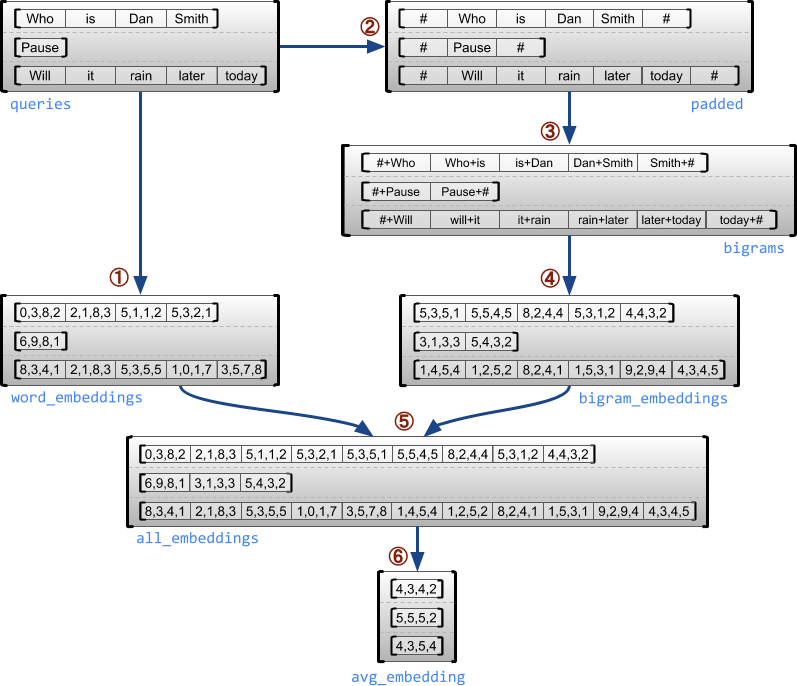Ragged and uniform dimensions

A ragged dimension is a dimension whose slices may have different lengths. For example, the inner (column) dimension of rt=[[3, 1, 4, 1], [], [5, 9, 2], , []] is ragged, since the column slices (rt[0, :], ..., rt[4, :]) have different lengths. Dimensions whose slices all have the same length are called uniform dimensions.

The outermost dimension of a ragged tensor is always uniform, since it consists of a single slice (and, therefore, there is no possibility for differing slice lengths). The remaining dimensions may be either ragged or uniform. For example, you may store the word embeddings for each word in a batch of sentences using a ragged tensor with shape [num_sentences, (num_words), embedding_size], where the parentheses around (num_words) indicate that the dimension is ragged.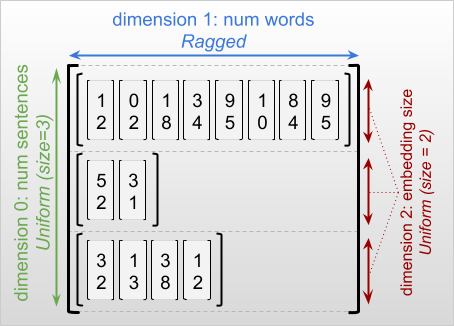Ragged tensors may have multiple ragged dimensions. For example, you could store a batch of structured text documents using a tensor with shape [num_documents, (num_paragraphs), (num_sentences), (num_words)] (where again parentheses are used to indicate ragged dimensions).

As with tf.Tensor, the rank of a ragged tensor is its total number of dimensions (including both ragged and uniform dimensions). A potentially ragged tensor is a value that might be either a tf.Tensor or a tf.RaggedTensor.

When describing the shape of a RaggedTensor, ragged dimensions are conventionally indicated by enclosing them in parentheses. For example, as you saw above, the shape of a 3D RaggedTensor that stores word embeddings for each word in a batch of sentences can be written as [num_sentences, (num_words), embedding_size].

The RaggedTensor.shape attribute returns a tf.TensorShape for a ragged tensor where ragged dimensions have size None:

tf.ragged.constant([["Hi"], ["How", "are", "you"]]).shape
TensorShape([2, None])

The method tf.RaggedTensor.bounding_shape can be used to find a tight bounding shape for a given RaggedTensor:

print(tf.ragged.constant([["Hi"], ["How", "are", "you"]]).bounding_shape())
tf.Tensor([2 3], shape=(2,), dtype=int64)

Ragged vs sparse

A ragged tensor should not be thought of as a type of sparse tensor. In particular, sparse tensors are efficient encodings for tf.Tensor that model the same data in a compact format; but ragged tensor is an extension to tf.Tensor that models an expanded class of data. This difference is crucial when defining operations:

• Applying an op to a sparse or dense tensor should always give the same result.
• Applying an op to a ragged or sparse tensor may give different results.

As an illustrative example, consider how array operations such as concat, stack, and tile are defined for ragged vs. sparse tensors. Concatenating ragged tensors joins each row to form a single row with the combined length:ragged_x = tf.ragged.constant([["John"], ["a", "big", "dog"], ["my", "cat"]])
ragged_y = tf.ragged.constant([["fell", "asleep"], ["barked"], ["is", "fuzzy"]])
print(tf.concat([ragged_x, ragged_y], axis=1))
<tf.RaggedTensor [[b'John', b'fell', b'asleep'], [b'a', b'big', b'dog', b'barked'], [b'my', b'cat', b'is', b'fuzzy']]>

However, concatenating sparse tensors is equivalent to concatenating the corresponding dense tensors, as illustrated by the following example (where Ø indicates missing values):sparse_x = ragged_x.to_sparse()
sparse_y = ragged_y.to_sparse()
sparse_result = tf.sparse.concat(sp_inputs=[sparse_x, sparse_y], axis=1)
print(tf.sparse.to_dense(sparse_result, ''))
tf.Tensor(
[[b'John' b'' b'' b'fell' b'asleep']
[b'a' b'big' b'dog' b'barked' b'']
[b'my' b'cat' b'' b'is' b'fuzzy']], shape=(3, 5), dtype=string)

For another example of why this distinction is important, consider the definition of “the mean value of each row” for an op such as tf.reduce_mean. For a ragged tensor, the mean value for a row is the sum of the row’s values divided by the row’s width. But for a sparse tensor, the mean value for a row is the sum of the row’s values divided by the sparse tensor’s overall width (which is greater than or equal to the width of the longest row).

TensorFlow APIs

Keras

tf.keras is TensorFlow's high-level API for building and training deep learning models. Ragged tensors may be passed as inputs to a Keras model by setting ragged=True on tf.keras.Input or tf.keras.layers.InputLayer. Ragged tensors may also be passed between Keras layers, and returned by Keras models. The following example shows a toy LSTM model that is trained using ragged tensors.

# Task: predict whether each sentence is a question or not.
sentences = tf.constant(
['What makes you think she is a witch?',
'She turned me into a newt.',
'A newt?',
'Well, I got better.'])
is_question = tf.constant([True, False, True, False])

# Preprocess the input strings.
hash_buckets = 1000
words = tf.strings.split(sentences, ' ')
hashed_words = tf.strings.to_hash_bucket_fast(words, hash_buckets)

# Build the Keras model.
keras_model = tf.keras.Sequential([
tf.keras.layers.Input(shape=[None], dtype=tf.int64, ragged=True),
tf.keras.layers.Embedding(hash_buckets, 16),
tf.keras.layers.LSTM(32, use_bias=False),
tf.keras.layers.Dense(32),
tf.keras.layers.Activation(tf.nn.relu),
tf.keras.layers.Dense(1)
])

keras_model.compile(loss='binary_crossentropy', optimizer='rmsprop')
keras_model.fit(hashed_words, is_question, epochs=5)
print(keras_model.predict(hashed_words))
WARNING:tensorflow:Layer lstm will not use cuDNN kernels since it doesn't meet the criteria. It will use a generic GPU kernel as fallback when running on GPU.
Epoch 1/5
/tmpfs/src/tf_docs_env/lib/python3.7/site-packages/tensorflow/python/framework/indexed_slices.py:449: UserWarning: Converting sparse IndexedSlices(IndexedSlices(indices=Tensor("gradient_tape/sequential/lstm/RaggedToTensor/boolean_mask_1/GatherV2:0", shape=(None,), dtype=int32), values=Tensor("gradient_tape/sequential/lstm/RaggedToTensor/boolean_mask/GatherV2:0", shape=(None, 16), dtype=float32), dense_shape=Tensor("gradient_tape/sequential/lstm/RaggedToTensor/Shape:0", shape=(2,), dtype=int32))) to a dense Tensor of unknown shape. This may consume a large amount of memory.
"shape. This may consume a large amount of memory." % value)
1/1 [==============================] - 2s 2s/step - loss: 3.1269
Epoch 2/5
1/1 [==============================] - 0s 18ms/step - loss: 2.1197
Epoch 3/5
1/1 [==============================] - 0s 19ms/step - loss: 2.0196
Epoch 4/5
1/1 [==============================] - 0s 20ms/step - loss: 1.9371
Epoch 5/5
1/1 [==============================] - 0s 18ms/step - loss: 1.8857
[[0.02800461]
[0.00945962]
[0.02283431]
[0.00252927]]

tf.Example

tf.Example is a standard protobuf encoding for TensorFlow data. Data encoded with tf.Examples often includes variable-length features. For example, the following code defines a batch of four tf.Example messages with different feature lengths:

def build_tf_example(s):
return pbtext.Merge(s, tf.train.Example()).SerializeToString()

example_batch = [
build_tf_example(r'''
features {
feature {key: "colors" value {bytes_list {value: ["red", "blue"]} } }
feature {key: "lengths" value {int64_list {value: } } } }'''),
build_tf_example(r'''
features {
feature {key: "colors" value {bytes_list {value: ["orange"]} } }
feature {key: "lengths" value {int64_list {value: []} } } }'''),
build_tf_example(r'''
features {
feature {key: "colors" value {bytes_list {value: ["black", "yellow"]} } }
feature {key: "lengths" value {int64_list {value: [1, 3]} } } }'''),
build_tf_example(r'''
features {
feature {key: "colors" value {bytes_list {value: ["green"]} } }
feature {key: "lengths" value {int64_list {value: [3, 5, 2]} } } }''')]

You can parse this encoded data using tf.io.parse_example, which takes a tensor of serialized strings and a feature specification dictionary, and returns a dictionary mapping feature names to tensors. To read the variable-length features into ragged tensors, you simply use tf.io.RaggedFeature in the feature specification dictionary:

feature_specification = {
'colors': tf.io.RaggedFeature(tf.string),
'lengths': tf.io.RaggedFeature(tf.int64),
}
feature_tensors = tf.io.parse_example(example_batch, feature_specification)
for name, value in feature_tensors.items():
print("{}={}".format(name, value))
colors=<tf.RaggedTensor [[b'red', b'blue'], [b'orange'], [b'black', b'yellow'], [b'green']]>
lengths=<tf.RaggedTensor [, [], [1, 3], [3, 5, 2]]>

tf.io.RaggedFeature can also be used to read features with multiple ragged dimensions. For details, refer to the API documentation.

Datasets

tf.data is an API that enables you to build complex input pipelines from simple, reusable pieces. Its core data structure is tf.data.Dataset, which represents a sequence of elements, in which each element consists of one or more components.

# Helper function used to print datasets in the examples below.
def print_dictionary_dataset(dataset):
for i, element in enumerate(dataset):
print("Element {}:".format(i))
for (feature_name, feature_value) in element.items():
print('{:>14} = {}'.format(feature_name, feature_value))

Building Datasets with ragged tensors

Datasets can be built from ragged tensors using the same methods that are used to build them from tf.Tensors or NumPy arrays, such as Dataset.from_tensor_slices:

dataset = tf.data.Dataset.from_tensor_slices(feature_tensors)
print_dictionary_dataset(dataset)
Element 0:
colors = [b'red' b'blue']
lengths = 
Element 1:
colors = [b'orange']
lengths = []
Element 2:
colors = [b'black' b'yellow']
lengths = [1 3]
Element 3:
colors = [b'green']
lengths = [3 5 2]

Batching and unbatching Datasets with ragged tensors

Datasets with ragged tensors can be batched (which combines n consecutive elements into a single elements) using the Dataset.batch method.

batched_dataset = dataset.batch(2)
print_dictionary_dataset(batched_dataset)
Element 0:
colors = <tf.RaggedTensor [[b'red', b'blue'], [b'orange']]>
lengths = <tf.RaggedTensor [, []]>
Element 1:
colors = <tf.RaggedTensor [[b'black', b'yellow'], [b'green']]>
lengths = <tf.RaggedTensor [[1, 3], [3, 5, 2]]>

Conversely, a batched dataset can be transformed into a flat dataset using Dataset.unbatch.

unbatched_dataset = batched_dataset.unbatch()
print_dictionary_dataset(unbatched_dataset)
Element 0:
colors = [b'red' b'blue']
lengths = 
Element 1:
colors = [b'orange']
lengths = []
Element 2:
colors = [b'black' b'yellow']
lengths = [1 3]
Element 3:
colors = [b'green']
lengths = [3 5 2]

Batching Datasets with variable-length non-ragged tensors

If you have a Dataset that contains non-ragged tensors, and tensor lengths vary across elements, then you can batch those non-ragged tensors into ragged tensors by applying the dense_to_ragged_batch transformation:

non_ragged_dataset = tf.data.Dataset.from_tensor_slices([1, 5, 3, 2, 8])
non_ragged_dataset = non_ragged_dataset.map(tf.range)
batched_non_ragged_dataset = non_ragged_dataset.apply(
tf.data.experimental.dense_to_ragged_batch(2))
for element in batched_non_ragged_dataset:
print(element)
<tf.RaggedTensor [, [0, 1, 2, 3, 4]]>
<tf.RaggedTensor [[0, 1, 2], [0, 1]]>
<tf.RaggedTensor [[0, 1, 2, 3, 4, 5, 6, 7]]>

Transforming Datasets with ragged tensors

You can also create or transform ragged tensors in Datasets using Dataset.map:

def transform_lengths(features):
return {
'mean_length': tf.math.reduce_mean(features['lengths']),
'length_ranges': tf.ragged.range(features['lengths'])}
transformed_dataset = dataset.map(transform_lengths)
print_dictionary_dataset(transformed_dataset)
Element 0:
mean_length = 7
length_ranges = <tf.RaggedTensor [[0, 1, 2, 3, 4, 5, 6]]>
Element 1:
mean_length = 0
length_ranges = <tf.RaggedTensor []>
Element 2:
mean_length = 2
length_ranges = <tf.RaggedTensor [, [0, 1, 2]]>
Element 3:
mean_length = 3
length_ranges = <tf.RaggedTensor [[0, 1, 2], [0, 1, 2, 3, 4], [0, 1]]>

tf.function

tf.function is a decorator that precomputes TensorFlow graphs for Python functions, which can substantially improve the performance of your TensorFlow code. Ragged tensors can be used transparently with @tf.function-decorated functions. For example, the following function works with both ragged and non-ragged tensors:

@tf.function
def make_palindrome(x, axis):
return tf.concat([x, tf.reverse(x, [axis])], axis)
make_palindrome(tf.constant([[1, 2], [3, 4], [5, 6]]), axis=1)
<tf.Tensor: shape=(3, 4), dtype=int32, numpy=
array([[1, 2, 2, 1],
[3, 4, 4, 3],
[5, 6, 6, 5]], dtype=int32)>
make_palindrome(tf.ragged.constant([[1, 2], , [4, 5, 6]]), axis=1)
2021-09-22 20:36:51.018367: W tensorflow/core/grappler/optimizers/loop_optimizer.cc:907] Skipping loop optimization for Merge node with control input: RaggedConcat/assert_equal_1/Assert/AssertGuard/branch_executed/_9
<tf.RaggedTensor [[1, 2, 2, 1], [3, 3], [4, 5, 6, 6, 5, 4]]>

If you wish to explicitly specify the input_signature for the tf.function, then you can do so using tf.RaggedTensorSpec.

@tf.function(
input_signature=[tf.RaggedTensorSpec(shape=[None, None], dtype=tf.int32)])
def max_and_min(rt):
return (tf.math.reduce_max(rt, axis=-1), tf.math.reduce_min(rt, axis=-1))

max_and_min(tf.ragged.constant([[1, 2], , [4, 5, 6]]))
(<tf.Tensor: shape=(3,), dtype=int32, numpy=array([2, 3, 6], dtype=int32)>,
<tf.Tensor: shape=(3,), dtype=int32, numpy=array([1, 3, 4], dtype=int32)>)

Concrete functions

Concrete functions encapsulate individual traced graphs that are built by tf.function. Ragged tensors can be used transparently with concrete functions.

@tf.function
def increment(x):
return x + 1

rt = tf.ragged.constant([[1, 2], , [4, 5, 6]])
cf = increment.get_concrete_function(rt)
print(cf(rt))
<tf.RaggedTensor [[2, 3], , [5, 6, 7]]>

SavedModels

A SavedModel is a serialized TensorFlow program, including both weights and computation. It can be built from a Keras model or from a custom model. In either case, ragged tensors can be used transparently with the functions and methods defined by a SavedModel.

Example: saving a Keras model

import tempfile

keras_module_path = tempfile.mkdtemp()
tf.saved_model.save(keras_model, keras_module_path)
imported_model(hashed_words)
2021-09-22 20:36:52.069689: W tensorflow/python/util/util.cc:348] Sets are not currently considered sequences, but this may change in the future, so consider avoiding using them.
WARNING:absl:Function `_wrapped_model` contains input name(s) args_0 with unsupported characters which will be renamed to args_0_1 in the SavedModel.
INFO:tensorflow:Assets written to: /tmp/tmp114axtt7/assets
INFO:tensorflow:Assets written to: /tmp/tmp114axtt7/assets
<tf.Tensor: shape=(4, 1), dtype=float32, numpy=
array([[0.02800461],
[0.00945962],
[0.02283431],
[0.00252927]], dtype=float32)>

Example: saving a custom model

class CustomModule(tf.Module):
def __init__(self, variable_value):
super(CustomModule, self).__init__()
self.v = tf.Variable(variable_value)

@tf.function
def grow(self, x):
return x * self.v

module = CustomModule(100.0)

# Before saving a custom model, you must ensure that concrete functions are
# built for each input signature that you will need.
module.grow.get_concrete_function(tf.RaggedTensorSpec(shape=[None, None],
dtype=tf.float32))

custom_module_path = tempfile.mkdtemp()
tf.saved_model.save(module, custom_module_path)
imported_model.grow(tf.ragged.constant([[1.0, 4.0, 3.0], [2.0]]))
INFO:tensorflow:Assets written to: /tmp/tmpnn4u8dy5/assets
INFO:tensorflow:Assets written to: /tmp/tmpnn4u8dy5/assets
<tf.RaggedTensor [[100.0, 400.0, 300.0], [200.0]]>

The RaggedTensor class overloads the standard Python arithmetic and comparison operators, making it easy to perform basic elementwise math:

x = tf.ragged.constant([[1, 2], , [4, 5, 6]])
y = tf.ragged.constant([[1, 1], , [3, 3, 3]])
print(x + y)
<tf.RaggedTensor [[2, 3], , [7, 8, 9]]>

Since the overloaded operators perform elementwise computations, the inputs to all binary operations must have the same shape or be broadcastable to the same shape. In the simplest broadcasting case, a single scalar is combined elementwise with each value in a ragged tensor:

x = tf.ragged.constant([[1, 2], , [4, 5, 6]])
print(x + 3)
<tf.RaggedTensor [[4, 5], , [7, 8, 9]]>

For a discussion of more advanced cases, check the section on Broadcasting.

Ragged tensors overload the same set of operators as normal Tensors: the unary operators -, ~, and abs(); and the binary operators +, -, *, /, //, %, **, &, |, ^, ==, <, <=, >, and >=.

Indexing

Ragged tensors support Python-style indexing, including multidimensional indexing and slicing. The following examples demonstrate ragged tensor indexing with a 2D and a 3D ragged tensor.

Indexing examples: 2D ragged tensor

queries = tf.ragged.constant(
[['Who', 'is', 'George', 'Washington'],
['What', 'is', 'the', 'weather', 'tomorrow'],
['Goodnight']])
print(queries)                   # A single query
tf.Tensor([b'What' b'is' b'the' b'weather' b'tomorrow'], shape=(5,), dtype=string)
print(queries[1, 2])                # A single word
tf.Tensor(b'the', shape=(), dtype=string)
print(queries[1:])                  # Everything but the first row
<tf.RaggedTensor [[b'What', b'is', b'the', b'weather', b'tomorrow'], [b'Goodnight']]>
print(queries[:, :3])               # The first 3 words of each query
<tf.RaggedTensor [[b'Who', b'is', b'George'], [b'What', b'is', b'the'], [b'Goodnight']]>
print(queries[:, -2:])              # The last 2 words of each query
<tf.RaggedTensor [[b'George', b'Washington'], [b'weather', b'tomorrow'], [b'Goodnight']]>

Indexing examples: 3D ragged tensor

rt = tf.ragged.constant([[[1, 2, 3], ],
[, [], ],
[],
[[8, 9], ]])
print(rt)                        # Second row (2D RaggedTensor)
<tf.RaggedTensor [, [], ]>
print(rt[3, 0])                     # First element of fourth row (1D Tensor)
tf.Tensor([8 9], shape=(2,), dtype=int32)
print(rt[:, 1:3])                   # Items 1-3 of each row (3D RaggedTensor)
<tf.RaggedTensor [[], [[], ], [], []]>
print(rt[:, -1:])                   # Last item of each row (3D RaggedTensor)
<tf.RaggedTensor [[], [], [], []]>

RaggedTensors support multidimensional indexing and slicing with one restriction: indexing into a ragged dimension is not allowed. This case is problematic because the indicated value may exist in some rows but not others. In such cases, it's not obvious whether you should (1) raise an IndexError; (2) use a default value; or (3) skip that value and return a tensor with fewer rows than you started with. Following the guiding principles of Python ("In the face of ambiguity, refuse the temptation to guess"), this operation is currently disallowed.

Tensor type conversion

The RaggedTensor class defines methods that can be used to convert between RaggedTensors and tf.Tensors or tf.SparseTensors:

ragged_sentences = tf.ragged.constant([
['Hi'], ['Welcome', 'to', 'the', 'fair'], ['Have', 'fun']])
# RaggedTensor -> Tensor
print(ragged_sentences.to_tensor(default_value='', shape=[None, 10]))
tf.Tensor(
[[b'Hi' b'' b'' b'' b'' b'' b'' b'' b'' b'']
[b'Welcome' b'to' b'the' b'fair' b'' b'' b'' b'' b'' b'']
[b'Have' b'fun' b'' b'' b'' b'' b'' b'' b'' b'']], shape=(3, 10), dtype=string)
# Tensor -> RaggedTensor
x = [[1, 3, -1, -1], [2, -1, -1, -1], [4, 5, 8, 9]]
<tf.RaggedTensor [[1, 3], , [4, 5, 8, 9]]>
#RaggedTensor -> SparseTensor
print(ragged_sentences.to_sparse())
SparseTensor(indices=tf.Tensor(
[[0 0]
[1 0]
[1 1]
[1 2]
[1 3]
[2 0]
[2 1]], shape=(7, 2), dtype=int64), values=tf.Tensor([b'Hi' b'Welcome' b'to' b'the' b'fair' b'Have' b'fun'], shape=(7,), dtype=string), dense_shape=tf.Tensor([3 4], shape=(2,), dtype=int64))
# SparseTensor -> RaggedTensor
st = tf.SparseTensor(indices=[[0, 0], [2, 0], [2, 1]],
values=['a', 'b', 'c'],
dense_shape=[3, 3])
print(tf.RaggedTensor.from_sparse(st))
<tf.RaggedTensor [[b'a'], [], [b'b', b'c']]>

Evaluating ragged tensors

To access the values in a ragged tensor, you can:

1. Use tf.RaggedTensor.to_list to convert the ragged tensor to a nested Python list.
2. Use tf.RaggedTensor.numpy to convert the ragged tensor to a NumPy array whose values are nested NumPy arrays.
3. Decompose the ragged tensor into its components, using the tf.RaggedTensor.values and tf.RaggedTensor.row_splits properties, or row-paritioning methods such as tf.RaggedTensor.row_lengths and tf.RaggedTensor.value_rowids.
4. Use Python indexing to select values from the ragged tensor.
rt = tf.ragged.constant([[1, 2], [3, 4, 5], , [], ])
print("Python list:", rt.to_list())
print("NumPy array:", rt.numpy())
print("Values:", rt.values.numpy())
print("Splits:", rt.row_splits.numpy())
print("Indexed value:", rt.numpy())
Python list: [[1, 2], [3, 4, 5], , [], ]
NumPy array: [array([1, 2], dtype=int32) array([3, 4, 5], dtype=int32)
array(, dtype=int32) array([], dtype=int32) array(, dtype=int32)]
Values: [1 2 3 4 5 6 7]
Splits: [0 2 5 6 6 7]
Indexed value: [3 4 5]
/tmpfs/src/tf_docs_env/lib/python3.7/site-packages/tensorflow/python/ops/ragged/ragged_tensor.py:2063: VisibleDeprecationWarning: Creating an ndarray from ragged nested sequences (which is a list-or-tuple of lists-or-tuples-or ndarrays with different lengths or shapes) is deprecated. If you meant to do this, you must specify 'dtype=object' when creating the ndarray
return np.array(rows)

Broadcasting is the process of making tensors with different shapes have compatible shapes for elementwise operations. For more background on broadcasting, refer to:

The basic steps for broadcasting two inputs x and y to have compatible shapes are:

1. If x and y do not have the same number of dimensions, then add outer dimensions (with size 1) until they do.

2. For each dimension where x and y have different sizes:

• If x or y have size 1 in dimension d, then repeat its values across dimension d to match the other input's size.
• Otherwise, raise an exception (x and y are not broadcast compatible).

Where the size of a tensor in a uniform dimension is a single number (the size of slices across that dimension); and the size of a tensor in a ragged dimension is a list of slice lengths (for all slices across that dimension).

# x       (2D ragged):  2 x (num_rows)
# y       (scalar)
# result  (2D ragged):  2 x (num_rows)
x = tf.ragged.constant([[1, 2], ])
y = 3
print(x + y)
<tf.RaggedTensor [[4, 5], ]>
# x         (2d ragged):  3 x (num_rows)
# y         (2d tensor):  3 x          1
# Result    (2d ragged):  3 x (num_rows)
x = tf.ragged.constant(
[[10, 87, 12],
[19, 53],
[12, 32]])
y = [, , ]
print(x + y)
<tf.RaggedTensor [[1010, 1087, 1012], [2019, 2053], [3012, 3032]]>
# x      (3d ragged):  2 x (r1) x 2
# y      (2d ragged):         1 x 1
# Result (3d ragged):  2 x (r1) x 2
x = tf.ragged.constant(
[[[1, 2], [3, 4], [5, 6]],
[[7, 8]]],
ragged_rank=1)
y = tf.constant([])
print(x + y)
<tf.RaggedTensor [[[11, 12], [13, 14], [15, 16]], [[17, 18]]]>
# x      (3d ragged):  2 x (r1) x (r2) x 1
# y      (1d tensor):                    3
# Result (3d ragged):  2 x (r1) x (r2) x 3
x = tf.ragged.constant(
[
[
[, ],
[],
[],
[],
],
[
[, ],
[]
]
],
ragged_rank=2)
y = tf.constant([10, 20, 30])
print(x + y)
<tf.RaggedTensor [[[[11, 21, 31], [12, 22, 32]], [], [[13, 23, 33]], [[14, 24, 34]]], [[[15, 25, 35], [16, 26, 36]], [[17, 27, 37]]]]>

Here are some examples of shapes that do not broadcast:

# x      (2d ragged): 3 x (r1)
# y      (2d tensor): 3 x    4  # trailing dimensions do not match
x = tf.ragged.constant([[1, 2], [3, 4, 5, 6], ])
y = tf.constant([[1, 2, 3, 4], [5, 6, 7, 8], [9, 10, 11, 12]])
try:
x + y
except tf.errors.InvalidArgumentError as exception:
print(exception)
Expected 'tf.Tensor(False, shape=(), dtype=bool)' to be true. Summarized data: b'Unable to broadcast: dimension size mismatch in dimension'
1
b'lengths='
4
b'dim_size='
2, 4, 1
# x      (2d ragged): 3 x (r1)
# y      (2d ragged): 3 x (r2)  # ragged dimensions do not match.
x = tf.ragged.constant([[1, 2, 3], , [5, 6]])
y = tf.ragged.constant([[10, 20], [30, 40], ])
try:
x + y
except tf.errors.InvalidArgumentError as exception:
print(exception)
Expected 'tf.Tensor(False, shape=(), dtype=bool)' to be true. Summarized data: b'Unable to broadcast: dimension size mismatch in dimension'
1
b'lengths='
2, 2, 1
b'dim_size='
3, 1, 2
# x      (3d ragged): 3 x (r1) x 2
# y      (3d ragged): 3 x (r1) x 3  # trailing dimensions do not match
x = tf.ragged.constant([[[1, 2], [3, 4], [5, 6]],
[[7, 8], [9, 10]]])
y = tf.ragged.constant([[[1, 2, 0], [3, 4, 0], [5, 6, 0]],
[[7, 8, 0], [9, 10, 0]]])
try:
x + y
except tf.errors.InvalidArgumentError as exception:
print(exception)
Expected 'tf.Tensor(False, shape=(), dtype=bool)' to be true. Summarized data: b'Unable to broadcast: dimension size mismatch in dimension'
2
b'lengths='
3, 3, 3, 3, 3
b'dim_size='
2, 2, 2, 2, 2

RaggedTensor encoding

Ragged tensors are encoded using the RaggedTensor class. Internally, each RaggedTensor consists of:

• A values tensor, which concatenates the variable-length rows into a flattened list.
• A row_partition, which indicates how those flattened values are divided into rows.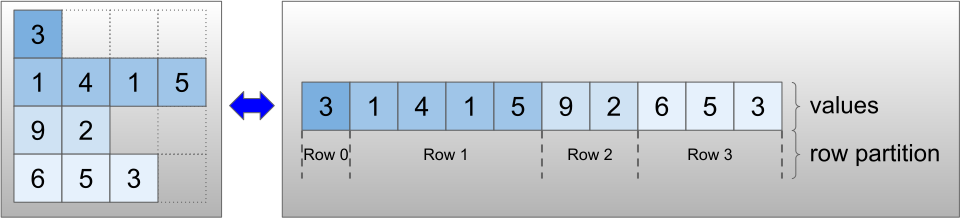The row_partition can be stored using four different encodings:

• row_splits is an integer vector specifying the split points between rows.
• value_rowids is an integer vector specifying the row index for each value.
• row_lengths is an integer vector specifying the length of each row.
• uniform_row_length is an integer scalar specifying a single length for all rows.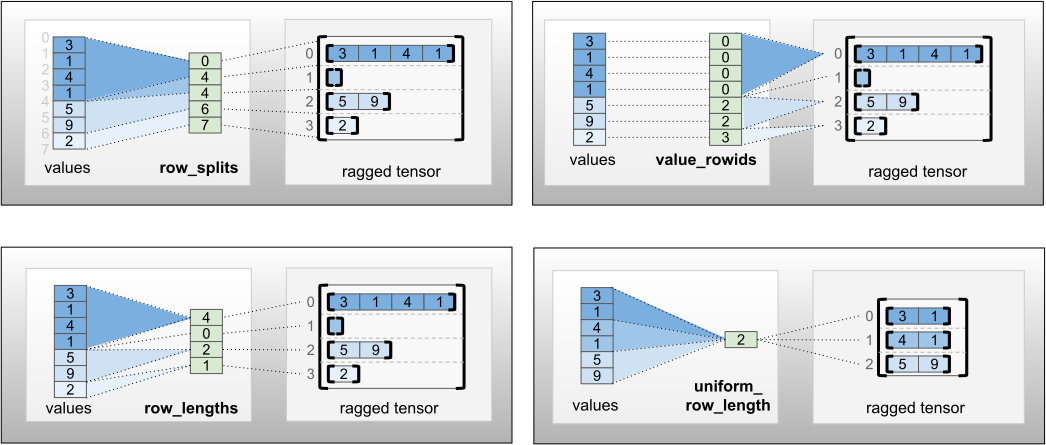An integer scalar nrows can also be included in the row_partition encoding to account for empty trailing rows with value_rowids or empty rows with uniform_row_length.

rt = tf.RaggedTensor.from_row_splits(
values=[3, 1, 4, 1, 5, 9, 2],
row_splits=[0, 4, 4, 6, 7])
print(rt)
<tf.RaggedTensor [[3, 1, 4, 1], [], [5, 9], ]>

The choice of which encoding to use for row partitions is managed internally by ragged tensors to improve efficiency in some contexts. In particular, some of the advantages and disadvantages of the different row-partitioning schemes are:

• Efficient indexing: The row_splits encoding enables constant-time indexing and slicing into ragged tensors.
• Efficient concatenation: The row_lengths encoding is more efficient when concatenating ragged tensors, since row lengths do not change when two tensors are concatenated together.
• Small encoding size: The value_rowids encoding is more efficient when storing ragged tensors that have a large number of empty rows, since the size of the tensor depends only on the total number of values. On the other hand, the row_splits and row_lengths encodings are more efficient when storing ragged tensors with longer rows, since they require only one scalar value for each row.
• Compatibility: The value_rowids scheme matches the segmentation format used by operations, such as tf.segment_sum. The row_limits scheme matches the format used by ops such as tf.sequence_mask.
• Uniform dimensions: As discussed below, the uniform_row_length encoding is used to encode ragged tensors with uniform dimensions.

Multiple ragged dimensions

A ragged tensor with multiple ragged dimensions is encoded by using a nested RaggedTensor for the values tensor. Each nested RaggedTensor adds a single ragged dimension.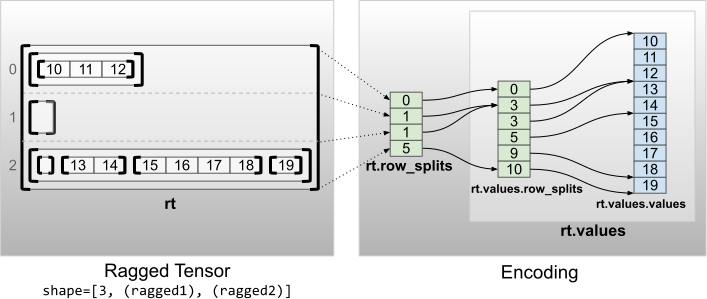rt = tf.RaggedTensor.from_row_splits(
values=tf.RaggedTensor.from_row_splits(
values=[10, 11, 12, 13, 14, 15, 16, 17, 18, 19],
row_splits=[0, 3, 3, 5, 9, 10]),
row_splits=[0, 1, 1, 5])
print(rt)
print("Shape: {}".format(rt.shape))
print("Number of partitioned dimensions: {}".format(rt.ragged_rank))
<tf.RaggedTensor [[[10, 11, 12]], [], [[], [13, 14], [15, 16, 17, 18], ]]>
Shape: (3, None, None)
Number of partitioned dimensions: 2

The factory function tf.RaggedTensor.from_nested_row_splits may be used to construct a RaggedTensor with multiple ragged dimensions directly by providing a list of row_splits tensors:

rt = tf.RaggedTensor.from_nested_row_splits(
flat_values=[10, 11, 12, 13, 14, 15, 16, 17, 18, 19],
nested_row_splits=([0, 1, 1, 5], [0, 3, 3, 5, 9, 10]))
print(rt)
<tf.RaggedTensor [[[10, 11, 12]], [], [[], [13, 14], [15, 16, 17, 18], ]]>

Ragged rank and flat values

A ragged tensor's ragged rank is the number of times that the underlying values tensor has been partitioned (i.e. the nesting depth of RaggedTensor objects). The innermost values tensor is known as its flat_values. In the following example, conversations has ragged_rank=3, and its flat_values is a 1D Tensor with 24 strings:

# shape = [batch, (paragraph), (sentence), (word)]
conversations = tf.ragged.constant(
[[[["I", "like", "ragged", "tensors."]],
[["Oh", "yeah?"], ["What", "can", "you", "use", "them", "for?"]],
[["Processing", "variable", "length", "data!"]]],
[[["I", "like", "cheese."], ["Do", "you?"]],
[["Yes."], ["I", "do."]]]])
conversations.shape
TensorShape([2, None, None, None])
assert conversations.ragged_rank == len(conversations.nested_row_splits)
conversations.ragged_rank  # Number of partitioned dimensions.
3
conversations.flat_values.numpy()
array([b'I', b'like', b'ragged', b'tensors.', b'Oh', b'yeah?', b'What',
b'can', b'you', b'use', b'them', b'for?', b'Processing',
b'variable', b'length', b'data!', b'I', b'like', b'cheese.', b'Do',
b'you?', b'Yes.', b'I', b'do.'], dtype=object)

Uniform inner dimensions

Ragged tensors with uniform inner dimensions are encoded by using a multidimensional tf.Tensor for the flat_values (i.e., the innermost values).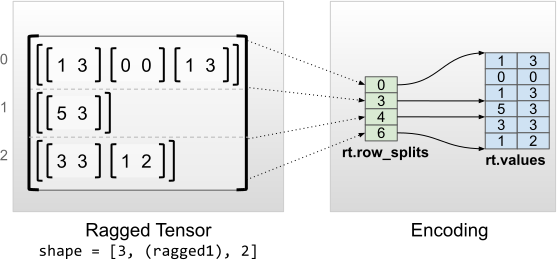rt = tf.RaggedTensor.from_row_splits(
values=[[1, 3], [0, 0], [1, 3], [5, 3], [3, 3], [1, 2]],
row_splits=[0, 3, 4, 6])
print(rt)
print("Shape: {}".format(rt.shape))
print("Number of partitioned dimensions: {}".format(rt.ragged_rank))
print("Flat values shape: {}".format(rt.flat_values.shape))
print("Flat values:\n{}".format(rt.flat_values))
<tf.RaggedTensor [[[1, 3], [0, 0], [1, 3]], [[5, 3]], [[3, 3], [1, 2]]]>
Shape: (3, None, 2)
Number of partitioned dimensions: 1
Flat values shape: (6, 2)
Flat values:
[[1 3]
[0 0]
[1 3]
[5 3]
[3 3]
[1 2]]

Uniform non-inner dimensions

Ragged tensors with uniform non-inner dimensions are encoded by partitioning rows with uniform_row_length.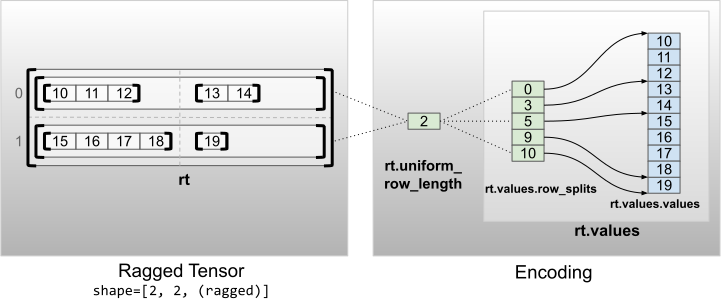rt = tf.RaggedTensor.from_uniform_row_length(
values=tf.RaggedTensor.from_row_splits(
values=[10, 11, 12, 13, 14, 15, 16, 17, 18, 19],
row_splits=[0, 3, 5, 9, 10]),
uniform_row_length=2)
print(rt)
print("Shape: {}".format(rt.shape))
print("Number of partitioned dimensions: {}".format(rt.ragged_rank))
<tf.RaggedTensor [[[10, 11, 12], [13, 14]], [[15, 16, 17, 18], ]]>
Shape: (2, 2, None)
Number of partitioned dimensions: 2
[{ "type": "thumb-down", "id": "missingTheInformationINeed", "label":"Missing the information I need" },{ "type": "thumb-down", "id": "tooComplicatedTooManySteps", "label":"Too complicated / too many steps" },{ "type": "thumb-down", "id": "outOfDate", "label":"Out of date" },{ "type": "thumb-down", "id": "samplesCodeIssue", "label":"Samples / code issue" },{ "type": "thumb-down", "id": "otherDown", "label":"Other" }]
[{ "type": "thumb-up", "id": "easyToUnderstand", "label":"Easy to understand" },{ "type": "thumb-up", "id": "solvedMyProblem", "label":"Solved my problem" },{ "type": "thumb-up", "id": "otherUp", "label":"Other" }]